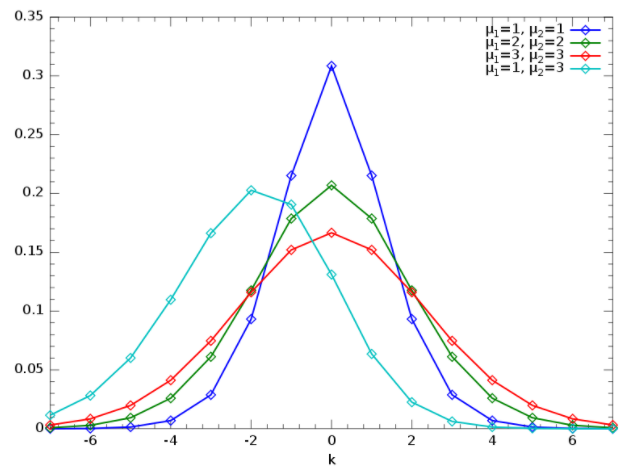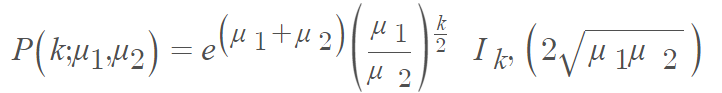# Skellam Distribution (Poisson Difference Distribution)

Share on

The Skellam distribution (also called the Poisson difference distribution) is a discrete probability distribution that shows differences between counts. It is derived from differences between Poisson random variables (i.e., two random variables that originate from a Poisson distribution) . Although the distribution is usually used for independent random variables, it can be applied to a special case of dependent variables when the two variables have a common additive random contribution — one that is canceled by differencing .

The distribution, first formulated by Skellam in 1946 , is used in a wide number of applications including:

• Image detection and denoising ,
• Showing the spread of points in sports, where points are equal (e.g., hockey, soccer) ,
• Studying treatment effects ,
• Modeling noise in PET imaging .

## Skellam Distribution PMFExamples of the probability mass function for different values of the Skellam distribution.

The Skellam distribution, which returns values between (-∞, ∞), is a function of the difference k between two Poisson random variables. It is expressed by the probability mass function (PMF) as :Where:

## References

PMF Examples image Krishnavedala, CC0, via Wikimedia Commons.
 Sellers, K. A distribution describing common differences in count data containing common disperson levels. Advanced and Applications in Statistical Science. Volume 7, Issue 3, March-April 2012, Pages 35-46. Mill Publications.
 Karlis, D. and Ntzoufras, I. (2003) “Analysis of sports data using bivariate Poisson models“. Journal of the Royal Statistical Society, Series D, 52 (3), 381–393. doi:10.1111/1467-9884.00366
 Skellam, J. G. (1946) “The frequency distribution of the difference between two Poisson variates belonging to different populations”. Journal of the Royal Statistical Society, Series A, 109 (3), 296.
 Hirakawa, K. et al. Wavelet-based Poisson Rate Estimation using the Skellam distribution, in C.A. Bouman et al. (Eds.). Proceedings of SPIE 7246 (2009).
 Karlis, D. & Ntoufras, I. Bayesian modelling of football outcomes: using the Skellam’s distribution for the goal difference. IMA J. Manag. Math 20(2) (2008), 133-145.
 Karlis, D. & Ntzoufras, I. Bayesian analysis of the differences of count data, Stat. Med. 25 (2006), 185-1905.
 M. Yavuz and J. A. Fessler. Maximum likelihood emission image reconstruction for randoms-precorrected pet scans. In IEEE Nuclear Science Symposium Conference Record, pages 15/229–15/233, 2000
 Hwang, Y. et al. Statistical background subtraction based on the exact per-pixel distributions. MVA2007 IAPR Conference on Machine Vision Applications, May 16-18, 2007, Tokyo, JAPAN

CITE THIS AS:
Stephanie Glen. "Skellam Distribution (Poisson Difference Distribution)" From StatisticsHowTo.com: Elementary Statistics for the rest of us! https://www.statisticshowto.com/skellam-distribution/
---------------------------------------------------------------------------Need help with a homework or test question? With Chegg Study, you can get step-by-step solutions to your questions from an expert in the field. Your first 30 minutes with a Chegg tutor is free!